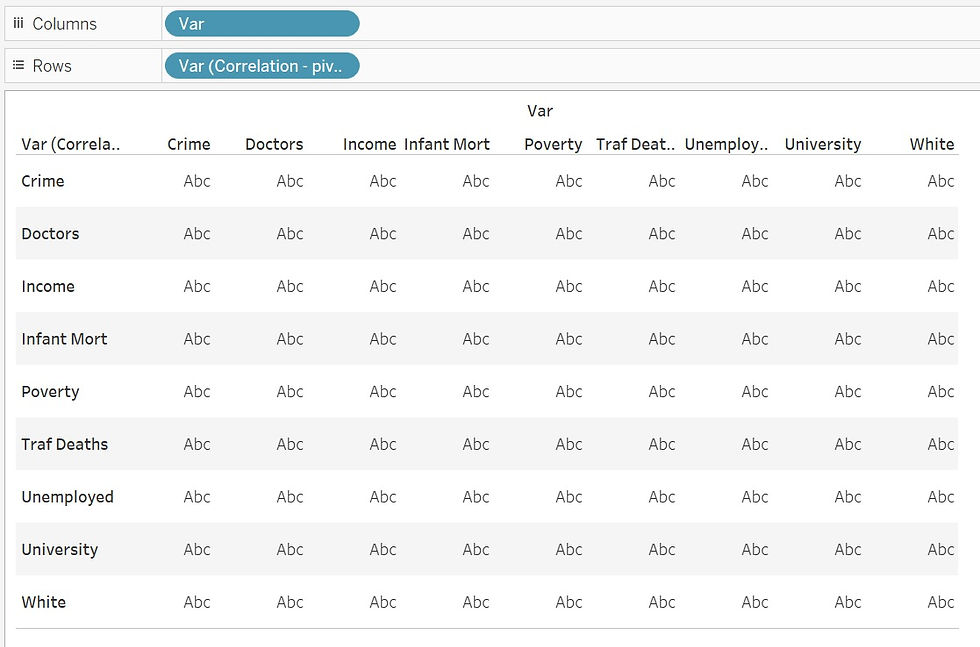top of page
Search
• Bernard K

# How to create correlation matrix/heatmap in Tableau### Introduction

Correlation is a statistic that measures the degree to which two variables move in coordination with each other. Where, if the two variables move in the same direction, then those variables are said to have a positive correlation. If they move in opposite directions, then they are said to have a negative correlation.

In Tableau, you can compute the correlation coefficient using these two functions.

• CORR () – Which returns the Pearson correlation coefficient of two expressions.

• WINDOW_CORR () – Which returns the Pearson correlation coefficient of two expressions within a window.

For example, using this sample data.I can compute the Pearson correlation coefficient for two variables, Crime and Poverty using the calculation below.Adding this calculation to the label shelf.Notice this value is equivalent to the square root of the R-squared value.(The R-squared value, is the square of the correlation)

### Creating correlation heatmap in Tableau

To create a correlation heatmap using the same data, first I will need to shape the data by converting it from crosstab to columnar format.

Export now pivoted data and connect it in Tableau.Add a second copy of the data set to the Tableau data source by relating the two using the common dimension ‘State’.On a new worksheet, add the dimension ‘Var’ from the two tables to create the view below.Compute the ‘Correlation Coefficient’ using the following calculation.Drag the above calculation to the color shelf and show labels.(This creates a correlation matrix – showing the correlation coefficients between variables in this sample data).

### Combining correlation matrix and scatter plot in a dashboard

Note, you can combine the correlation matrix and scatter plot to create a cool dashboard that allows you experience seamless interactions between the variables as well as access more statistics.### Conclusion

If you like the work we do and would like to work with us, drop us an email on our contacts page and we’ll reach out!